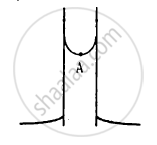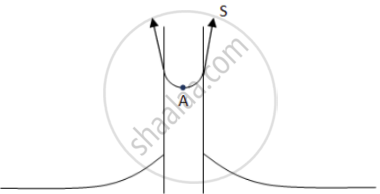Advertisement Remove all ads

# Figure Shows a Capillary Tube of Radius R Dipped into Water. If the Atmospheric Pressure Is P0, the Pressure at Point A Is - Physics

MCQ

Figure shows a capillary tube of radius r dipped into water. If the atmospheric pressure is P0, the pressure at point A is#### Options

• P0

• $P_0 + \frac{2S}{r}$

• $P_0 - \frac{2S}{r}$

• $P_0 - \frac{4S}{r}$

Advertisement Remove all ads

#### Solution$\text{ Here }:$

$\text{ Radius of the tube = r }$

$\text{ Net upward force due to surface tension = S }\text{ cos }\theta \times 2\pi r$

$\text{ Upward pressure }= \frac{\text{ S } cos \theta \times 2\pi r}{\pi r^2} = \frac{2Scos\theta}{r}$

$\text{ Net downward pressure due to atmosphere }= P_o$

$\Rightarrow \text{ Net pressure at A }= P_o - \frac{2Scos\theta}{r}$

$\text{ Since }\theta \text{ is small, }$

$\text{ cos }\theta \approx 1 .$

$\Rightarrow \text{ Net pressure }= P_o - \frac{2S}{r}$

Is there an error in this question or solution?
Advertisement Remove all ads

#### APPEARS IN

HC Verma Class 11, 12 Concepts of Physics 1
Chapter 14 Some Mechanical Properties of Matter
MCQ | Q 18 | Page 298
Advertisement Remove all ads

#### Video TutorialsVIEW ALL 

Advertisement Remove all ads
Share
Notifications

View all notifications

Forgot password?